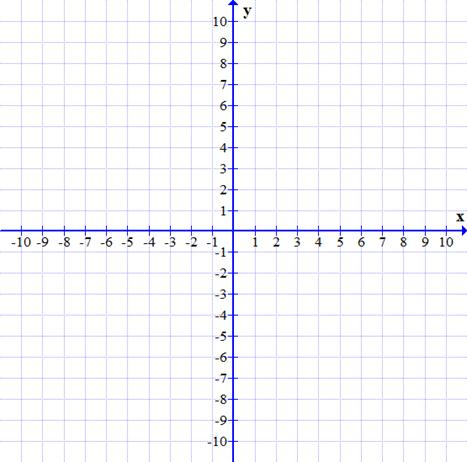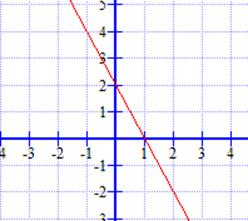### Sample Problem

Graph the functions 2x + y = 2 and 4x + 2y = 4.How many solutions does this system have?

#### SolutionGraphing these equations shows us that the lines coincide and thus, have an infinite number of solutions.